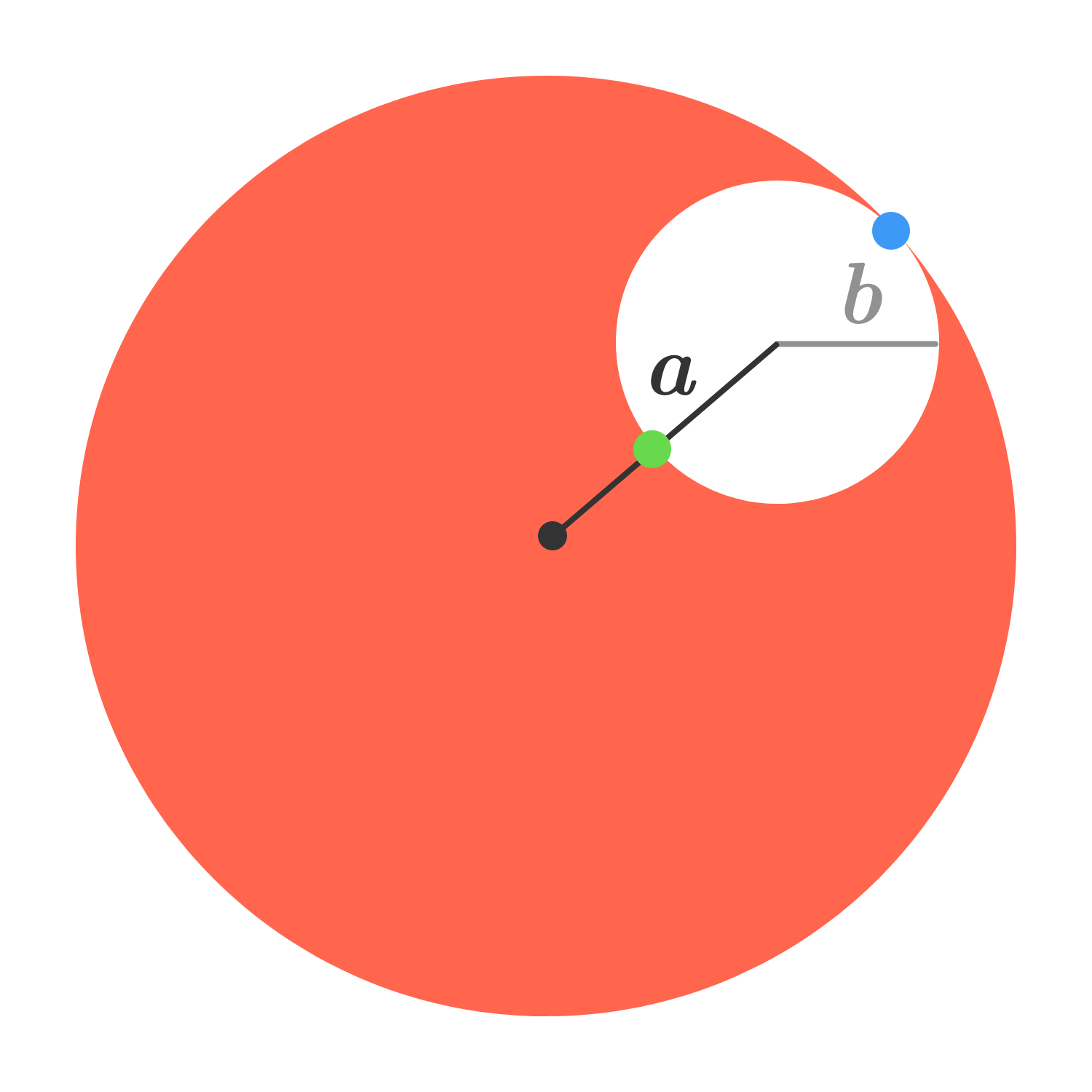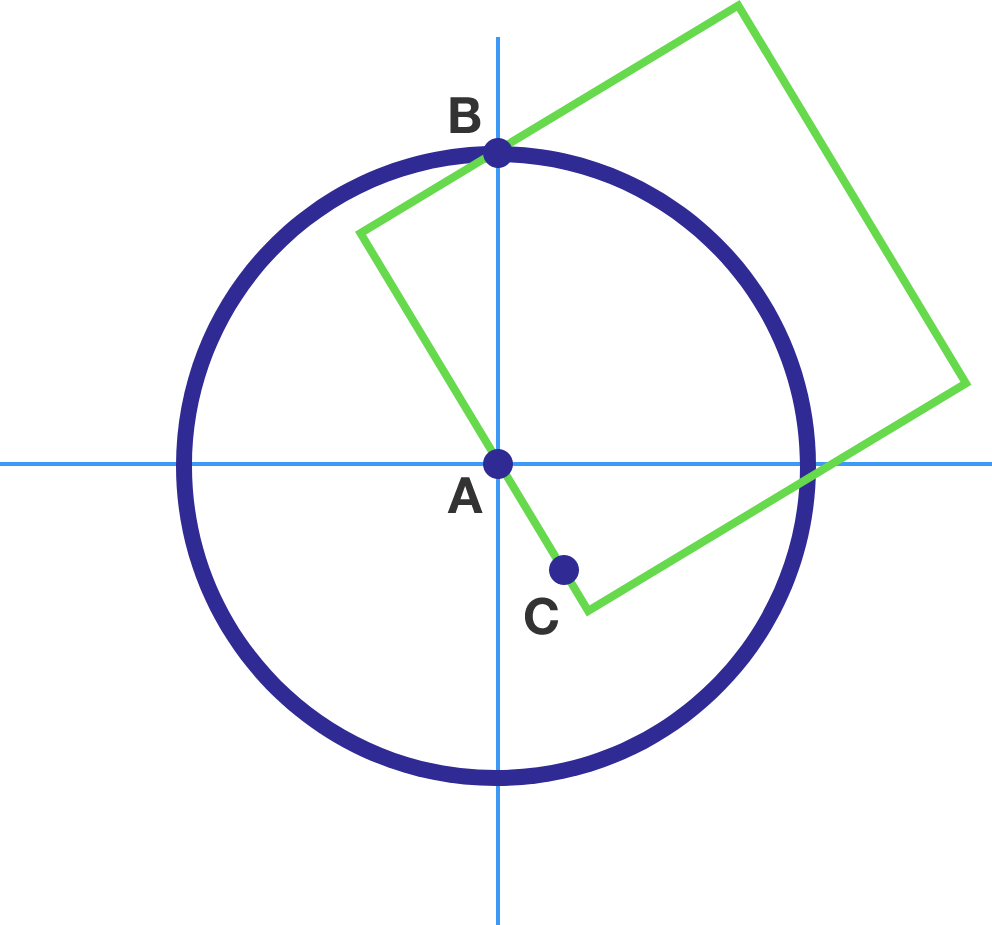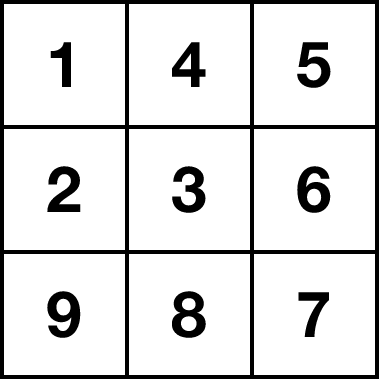# Problems of the Week

Contribute a problem

# 2017-05-29 AdvancedA sphere of uniform mass density $\rho$ and radius $a+b$ has a spherical hole of radius $b$ drilled a distance $a$ from its center. The gravitational field is measured at the far side of the hole (at the blue point) and is found to be $g_0.$

What will the field measure at the near side of the hole (at the green point)?

Let $x, y, z$ be real numbers satisfying $x+y+z=15$ and $xy+yz+zx=72$. Let the minimum value of $x$ be $S,$ and the maximum value $M$.

Find the value of $S+M.$

Consider two points: $A=(0,0)$ which is the center of a unit circle, and $B=(0,1)$ which lies on that unit circle. Now, you choose a third point $C$ inside the circle uniformly at random.What is the probability that you will be able to draw a square such that all three points $A, B, C$ lie on two adjacent sides of the square?

Please submit your answer to two decimal places.

We need to fill each cell in a $6\times6$ grid with a distinct integer from 1 to 36. There are two rules:

• Every pair of consecutive numbers are in adjacent cells that share an edge.
• Any two cells containing a multiple of 4 cannot share an edge nor a vertex.

Over all valid configurations, how many of the 36 cells could contain the number $36?$


Note: Below is a $3\times 3$ grid adhering to the rules above.$\large p^{3a+1}+p^a+1=b^p$

Find all solutions to the Diophantine equation above, where $a$ and $b$ are positive integers and $p$ is an odd prime, and enter your answer as $\sum (a+b+p)$.

×

Problem Loading...

Note Loading...

Set Loading...